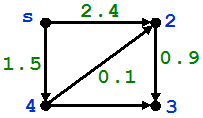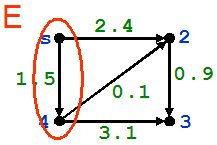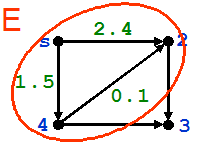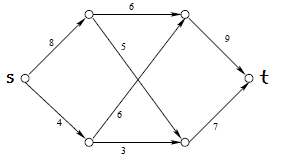# Backscatter

In this episode the Russian mafia is interested in e-mail spam to obtain sensible personal information of Bank customers. The mafia also threatens Don and his family.

## Routing

During the show, Charlie and Amita tried to find the ones who emptied Don's accounts by following the flow of information in the Internet. But how is the information in the Internet transmitted?  The process of selecting paths in a network to send date is called routing. There are many algorithms used to make this process "efficient" (depending on what one means by efficient) For instance, if one thinks of a network as a directed graph where there is a weight assigned to its edges (you can think of the weight of an edge (u,v) as being the number of seconds that it takes to transmit information between u and v) it is conceivable that we might be interested in finding the fastest way to provide information from a source to all the other vertices (routers) in the network.

There exist an algorithm to solve this problem: Dijkstra's algorithm. We describe this algorithm:Dijkstra's algorithm was invented by the Dutch computer scientist Edsger Wybe Dijkstra (May 11, 1930 – August 6, 2002)
Dijkstra's algorithm
INPUT:
A directed graph with one source s, and non-negative weights for every edge.
OUTPUT:  Finds a minimum-weight path between s and all the other vertices in the graph.
DESCRIPTION: Let d(v) denote the distance (length of shortest path) from s to v. For instance, in the example below,we have that d(s) = 0, d(2) = 1.6, d(3) = 2.5, d(4)=1.5.
The algorithm mantains a set E of "explored" vertices v for which we have determined the minimum-weight path between s and v. At the beginning, E = {s} (because d(s) = 0).

STEP 0 (initialization): For all vertices, set d(v)=w(s,v) (if (s,v) is not an edge, then set d(v) = infinity)
STEP 1: Find the vertex u that minimizes w(s,u), where w(s,u) is the weight of edge (s,u). Add u to E.
STEP 2: For each node v not in E such that (u,v) is and edge, if d(u) + w(u,v) < d(v), set d(v) = d(u) + w(u,v).
Now repeat steps 1 and 2 until all the vertices are in E.

For instance, for the graph above, the first iteration is depicted belowwhich yields d(s) = 0, d(2) = 2.4, d(3) = infinity, d(4)=1.5.
The second iteration, depicted below,yields d(s) = 0, d(2) = 1.6, d(3) = 4.6, d(4)=1.5.
The third iteration, depicted below,yields d(s) = 0, d(2) = 1.6, d(3) = 2.5, d(4)=1.5. After the third iteration, we include 3 to E, terminating the algorithm.
Activity 1
1. Find the minimum-weight paths from s to any other vertex in the network below2. We have assumed that the weights are non-negative, after all, it takes a positive amount of seconds to transmite a signal from one router to the other. Do you think the algorithm would work if negative weigths were allowed? (for instance, if the weights were the number of dollars that it costs to connect two routers, it might be the case that connecting two points actually generates a profit, which would be represented by a negative weight)

3. Dijkstra's algorithm is a greedy algorithm; that is, a procedure where at each step we select the optimal option based on local information and the information on the rest of the problem is not relevant (in Dijkstra's algorithm, the path that minimizes d(v)+w(u,v), where v is a neighbord of u. So vertices that are not in E and are not adjacent to u are not relevant). Can you think of a problem where the greedy algorithm does not work?

## Game Theory

In the episode Charlie tried to use game theory to understand Yuri Koverchenko's reasons to hack into Don's bank accounts. Here we briefly explain some ideas of game theory. Game Theory considers situations where at least two people (aka players) are involved. Each player's decisions influence the outcome of a certain event in a way which is spelled out in the rules of the game. Depending on which event actually occurs, the players receive a reward (which could be money). Usually the players will not agree on what event should occur, and thus have different goals when playing (like the FBI and the Russian mob). We now focus on matrix games, where one player wins what the others loose. Games like tick-tack-toe, bridge, poker, chess, go, and others can be put in the form of matrix games, although they are too complicated to be completely analyzed (otherwise, it would not be fun to play them!)

Consider the following game: There are two players, A and B. Player A is given a black 5 and a red 5, while player B is given a red 2, a red 3, and a black 5. The rules are the following: both players know what cards were given to the other; they pick one card and show it to each other at the same time. If both players pick cards of the same color, player B must pay player A the difference between the numerical values of the cards. On the other hand, if they pick cards of different colors, player A pays the (positive) difference between the numerical values of the cards.

We can summarise the payout rules with the following table

 B chooses red 2 B chooses red 3 B chooses black 5 A chooses red 5 (3,-3) (2,-2) (0,0) A chooses black 5 (-3,3) (-2,2) (0,0)

where (a,b) means that player A gets a dollars (if a<0, player A owns B a dollars) and player B gets b dollars (if b<0, player B owns A b dollars).

One would expect the players to choose the option that yields the highest reward. In B's case, this is choosing red 2. But A could easily expect player B to do just that! On the other hand, it is more convenient for A to choose the red card, since then he cannot loose any money. Similarly, if B picks black 5, he won't win anything, but he won't loose either.

We have only considered games with two players where what one wins is what the other one looses. This kind of game is called zero-sum game. Of course we can consider matrices where this is not the case; that is, tables with entries (a,b), with a not being the negative of b. These games are more complicated.
Activity 2
1. Suppose you are player A, what card would you choose? What if you are B?

2. Considering the game given by the following table
 B B A (0,0) (-15,15) A (1,-1) (5,-5)

How do you think the players should move?